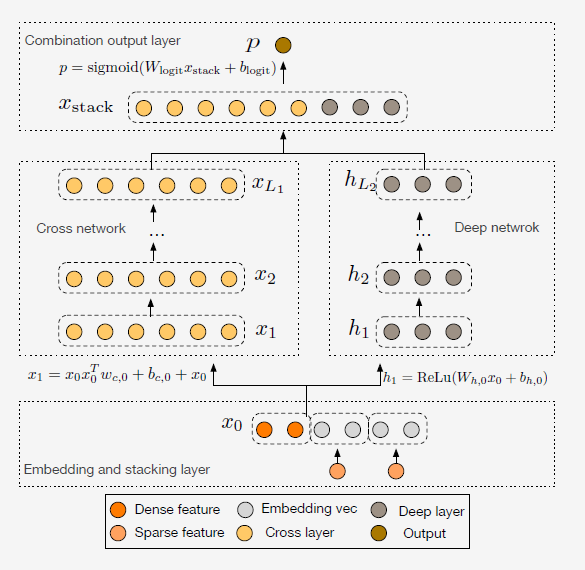# DCN¶

## Introduction¶

[paper]

Title: Deep & Cross Network for Ad Click Predictions

Authors: Ruoxi Wang, Bin Fu, Gang Fu, Mingliang Wang

Abstract: Feature engineering has been the key to the success of many prediction models. However, the process is nontrivial and oen requires manual feature engineering or exhaustive searching. DNNs are able to automatically learn feature interactions; however, they generate all the interactions implicitly, and are not necessarily efficient in learning all types of cross features. In this paper, we propose the Deep & Cross Network (DCN) which keeps the benefits of a DNN model, and beyond that, it introduces a novel cross network that is more efficient in learning certain bounded-degree feature interactions. In particular, DCN explicitly applies feature crossing at each layer, requires no manual feature engineering, and adds negligible extra complexity to the DNN model. Our experimental results have demonstrated its superiority over the state-of-art algorithms on the CTR prediction dataset and dense classification dataset, in terms of both model accuracy and memory usage.Model Hyper-Parameters:

• `embedding_size (int)` : The embedding size of features. Defaults to `10`.

• `mlp_hidden_size (list of int)` : The hidden size of MLP layers. Defaults to `[256,256,256]`.

• `cross_layer_num (int)` : The number of cross layers. Defaults to `6`.

• `reg_weight (float)` : The L2 regularization weight. Defaults to `2`.

• `dropout_prob (float)` : The dropout rate. Defaults to `0.2`.

A Running Example:

Write the following code to a python file, such as run.py

```from recbole.quick_start import run_recbole

run_recbole(model='DCN', dataset='ml-100k')
```

And then:

```python run.py
```

## Tuning Hyper Parameters¶

If you want to use `HyperTuning` to tune hyper parameters of this model, you can copy the following settings and name it as `hyper.test`.

```learning_rate choice [0.01,0.005,0.001,0.0005,0.0001]
dropout_prob choice [0.0,0.1,0.2,0.3,0.4,0.5]
mlp_hidden_size choice ['[64,64,64]','[128,128,128]','[256,256,256]','[512,512,512]','[1024, 1024]']
reg_weight choice [0.1,1,2,5,10]
cross_layer_num choice [3,4,5,6]
```

Note that we just provide these hyper parameter ranges for reference only, and we can not guarantee that they are the optimal range of this model.

Then, with the source code of RecBole (you can download it from GitHub), you can run the `run_hyper.py` to tuning:

```python run_hyper.py --model=[model_name] --dataset=[dataset_name] --config_files=[config_files_path] --params_file=hyper.test
```

For more details about Parameter Tuning, refer to Parameter Tuning.

If you want to change parameters, dataset or evaluation settings, take a look at